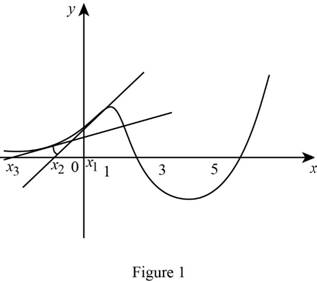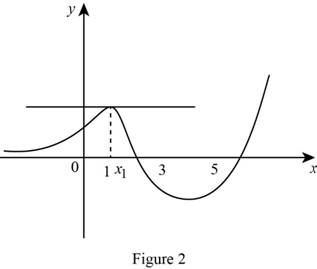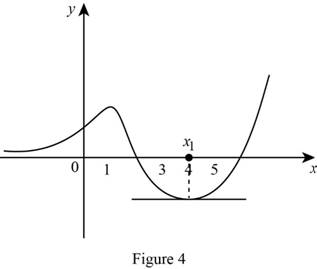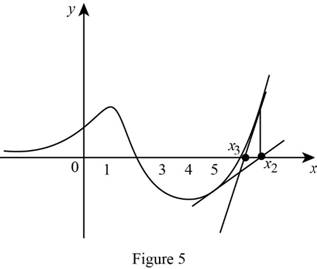# The graph of a tangent line at x 1 = 0 .### Single Variable Calculus: Concepts...

4th Edition
James Stewart
Publisher: Cengage Learning
ISBN: 9781337687805### Single Variable Calculus: Concepts...

4th Edition
James Stewart
Publisher: Cengage Learning
ISBN: 9781337687805

#### Solutions

Chapter 4.7, Problem 4E

(a)

To determine

## To sketch: The graph of a tangent line at x1=0.

Expert Solution

### Explanation of Solution

At first draw the tangent line at the point x1=0 and the tangent line intercept the x -axis.

Let the intercept point is x2 and the value of that point is negative.

Similarly draw the tangent line at the point x2 and the tangent line intercept the x-axis.

Let that intercept point is x3 and value of that point more negative than previous one.

Graph:Observation:

From Figure 1, observed that the point x1=0 is Newton’s method fail.

(b)

To determine

Expert Solution

### Explanation of Solution

At first draw the graph of tangent line at the point x1=1 and the tangent line intercept x-axis.

Let the intercept point is x2 and that is parallel to x-axis.

Graph:Observation:

From Figure 2 observed that the Newton’s method fail.

(c)

To determine

Expert Solution

### Explanation of Solution

At first draw the tangent line at the point x1=4 and the tangent line intercept the x -axis.

Let the intercept point is x2.

Graph:Observation:

From Figure 3, observed that the point x2=1 The Newton’s method fail.

(d)

To determine

Expert Solution

### Explanation of Solution

At first draw the graph of tangent line at the point x1=1 and the tangent line intercept x-axis.

Let the intercept point is x2 and that is parallel to x-axis.

Graph:Observation:

From Figure 4 observed that the Newton’s method fail.

(e)

To determine

Expert Solution

### Explanation of Solution

At first draw the tangent line at the point x1=5 and the tangent line intercept the x -axis.

Let the intercept point is x2.

Similarly draw the tangent line at the point x2 and the tangent line intercept the x-axis.

Let that intercept point x3.

Graph:Observation:

From Figure 5, observed that the point x1=5 is Newton’s method work.

### Have a homework question?

Subscribe to bartleby learn! Ask subject matter experts 30 homework questions each month. Plus, you’ll have access to millions of step-by-step textbook answers!